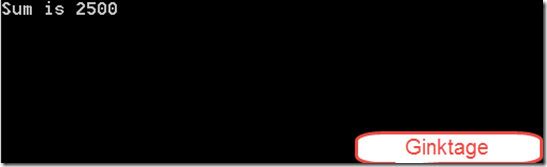# How to Find the Sum of odd numbers from 0 to 100 in F# ?

Recently , I started learning F# as hobby and started writing some simple programs . Today , I was trying out a sample program in F# which finds out the sum of odd numbers from 0 to 100 in F#.

# How to Find the Sum of odd numbers from 0 to 100 in F# ?

Below is a sample code snippet that does the work.

```// Ginktage - F# Program to find the sum of odd numbers from 0 to 100
open System
[<EntryPoint>]
let main argv =
let mutable sum = 0
for indexer=0 to 100 do
if indexer%2 <> 0 then
sum <- sum + indexer
printfn "Sum is %A" sum
// Printing the entered input